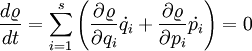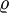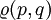Liouville's theorem$\frac{d\varrho}{dt}= \sum_{i=1}^{s} \left( \frac{\partial \varrho}{\partial q_i} \dot{q_i}+ \frac{\partial \varrho}{\partial p_i} \dot{p_i} \right) =0$
where$\varrho$ is a distribution function$\varrho(p,q)$, p is the generalised momenta and q are the generalised coordinates. With time a volume element can change shape, but phase points neither enter nor leave the volume.